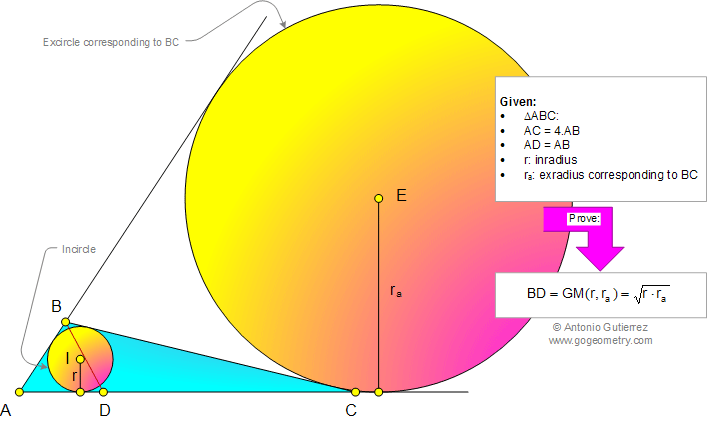# Geometry Problem 959. Triangle, Sides Ratio 4:1, Inradius, Exradius, Cevian, Mean Proportional, Geometric Mean

< PREVIOUS PROBLEM  |  NEXT PROBLEM >

 The figure below shows a triangle ABC so that AC = 4AB and AD = AB (D on AC). Prove that BD is the mean proportional between the inradius and the exradius corresponding to BC.Home | Geometry | Search | Problems | All Problems | Open Problems | Visual Index | 10 Problems | Problems Art GalleryArt | 951-960 | Triangles | Incircle | Excircle | Geometric Mean | Email | By Antonio Gutierrez